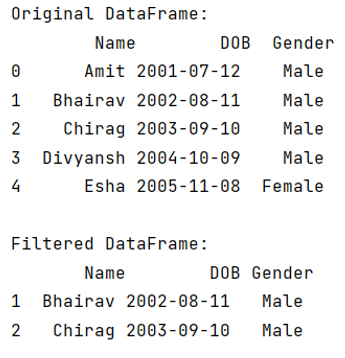# How to filter Pandas DataFrames on dates?

Given a DataFrame, we have to filter it on dates. By Pranit Sharma Last updated : September 20, 2023

Pandas is a special tool that allows us to perform complex manipulations of data effectively and efficiently. Inside pandas, we mostly deal with a dataset in the form of DataFrame. DataFrames are 2-dimensional data structures in pandas. DataFrames consist of rows, columns, and the data. The Data inside the DataFrame can be of any type.

Datetime is a library in python which is a collection of dates and times. Inside Datetime, we can access date and time in any format, but usually, the date is present in the format of "yy-mm-dd" and time is present in the format of "HH:MM:SS".

Here,

• yy means year
• mm means month
• dd means day
• HH means hours
• MM means minutes
• SS means seconds

## Filtering Pandas DataFrames on dates

For this purpose, we will first convert Date which is in string format into Date using the pandas.to_datetime() method and then we will select the data column to filter DataFrame on dates. The pandas.to_datetime() method is used to convert the string into the datetime format. When a CSV file is loaded or when a DataFrame is created then the date is created in string format, this method converts this string date into the correct format.

Syntax:

```pandas.to_datetime(
arg,
errors='raise',
dayfirst=False,
yearfirst=False,
utc=None,
format=None,
exact=True,
unit=None,
infer_datetime_format=False,
origin='unix',
cache=True
)
```

Parameter(s):

• It takes the string which has to be converted into the datetime format.
• It also takes some optional arguments like dayfirst, yearfirst, utc, format.

Let us understand with the help of an example.

## Python program to filter Pandas DataFrames on dates

```# Importing pandas package

import pandas as pd

# Creating a Dictionary
dict = {
'Name':['Amit','Bhairav','Chirag','Divyansh','Esha'],
'DOB':['07/12/2001','08/11/2002','09/10/2003','10/09/2004','11/08/2005'],
'Gender':['Male','Male','Male','Male','Female']
}

# Creating a DataFrame
df = pd.DataFrame(dict)

# Converting the column DOB value into datetime format
df['DOB']= pd.to_datetime(df['DOB'])

# Display DataFrame
print("Original DataFrame:\n",df,"\n")

# Filtering DataFrame
result = (df['DOB'] > '2002-08-01' ) & (df['DOB'] <= '2004-09-15')

filtered_df = df.loc[result]

# Display filtered data
print("Filtered DataFrame:\n",filtered_df)
```

### Output

The output of the above program is: